# Linear and logistic regression with RPosted by

This post is an analysis that applies linear and logistic regression on provided data with some health parameters of 2353 patiens who suffered surgeries. We try to discover the relation among some of the parameters and predict the probability of suffering an infection during the surgery.

data<-read.csv2("./data.csv",header = TRUE, sep = ",",stringsAsFactors = TRUE)

Let’s check the data.

str(data)
## 'data.frame':    2353 obs. of  16 variables:
##  $EDAD : int 59 65 69 70 79 55 56 65 70 80 ... ##$ SEXO    : Factor w/ 2 levels "mujer","varón": 1 1 2 1 1 1 1 1 1 1 ...
##  $PATOL : Factor w/ 4 levels "inflam","neo",..: 1 3 2 2 1 3 4 3 2 3 ... ##$ TIP_OPER: Factor w/ 4 levels "contam","limpia",..: 4 3 1 1 1 3 1 3 3 3 ...
##  $ALB : Factor w/ 8 levels "1","2","3","3.9",..: 5 5 5 NA NA 5 NA 3 5 3 ... ##$ HB      : int  13 12 13 14 8 12 13 11 8 12 ...
##  $HCTO : int 38 36 38 39 23 38 41 35 28 36 ... ##$ LEUCOS  : int  19090 6190 6360 8200 16940 4800 5550 5840 7400 4130 ...
##  $LINFOPCT: int 12 33 28 23 8 51 40 48 20 17 ... ##$ HEMAT   : int  4 4 4 5 3 4 5 4 4 4 ...
##  $GLUC : int 68 79 85 87 88 92 92 93 96 97 ... ##$ OBES    : Factor w/ 2 levels "no","si": 2 2 NA 1 NA 2 1 2 1 1 ...
##  $DESNUTR : Factor w/ 2 levels "no","si": 1 1 1 2 1 1 1 1 1 1 ... ##$ DIABETES: Factor w/ 2 levels "no","si": 2 2 2 2 2 2 2 2 2 2 ...
##  $INFEC : Factor w/ 2 levels "no","si": 2 1 2 1 2 2 2 1 1 1 ... ##$ GLUC_4  : Factor w/ 4 levels "(115-138]","<70",..: 2 4 4 4 4 4 4 4 4 4 ...

## Data cleaning

Let’s check if the data needs some cleaning.

Let’s check NA values.

colSums(is.na(data))
##     EDAD     SEXO    PATOL TIP_OPER      ALB       HB     HCTO   LEUCOS
##        2        0        0        0     1046        9        7       17
## LINFOPCT    HEMAT     GLUC     OBES  DESNUTR DIABETES    INFEC   GLUC_4
##       29      103        0      323        7        0        0        0
colSums(data=="")
##     EDAD     SEXO    PATOL TIP_OPER      ALB       HB     HCTO   LEUCOS
##       NA        0        0        0       NA       NA       NA       NA
## LINFOPCT    HEMAT     GLUC     OBES  DESNUTR DIABETES    INFEC   GLUC_4
##       NA       NA        0       NA       NA        0        0        0

We are going to delete the rows with any NA values, but ignoring ALB because there are many rows with a NA value in this variable, and we are not going to use it in this analysis.

data<- data[!is.na(data$EDAD),] data<- data[!is.na(data$HB),]
data<- data[!is.na(data$LEUCOS),] data<- data[!is.na(data$HEMAT),]
data<- data[!is.na(data$OBES),] data<- data[!is.na(data$DESNUTR),]

str(data)
## 'data.frame':    1936 obs. of  16 variables:
##  $EDAD : int 59 65 70 55 56 65 70 80 53 77 ... ##$ SEXO    : Factor w/ 2 levels "mujer","varón": 1 1 1 1 1 1 1 1 1 1 ...
##  $PATOL : Factor w/ 4 levels "inflam","neo",..: 1 3 2 3 4 3 2 3 2 2 ... ##$ TIP_OPER: Factor w/ 4 levels "contam","limpia",..: 4 3 1 3 1 3 3 3 3 3 ...
##  $ALB : Factor w/ 8 levels "1","2","3","3.9",..: 5 5 NA 5 NA 3 5 3 5 3 ... ##$ HB      : int  13 12 14 12 13 11 8 12 10 12 ...
##  $HCTO : int 38 36 39 38 41 35 28 36 29 37 ... ##$ LEUCOS  : int  19090 6190 8200 4800 5550 5840 7400 4130 4810 9590 ...
##  $LINFOPCT: int 12 33 23 51 40 48 20 17 20 13 ... ##$ HEMAT   : int  4 4 5 4 5 4 4 4 3 4 ...
##  $GLUC : int 68 79 87 92 92 93 96 97 99 99 ... ##$ OBES    : Factor w/ 2 levels "no","si": 2 2 1 2 1 2 1 1 1 1 ...
##  $DESNUTR : Factor w/ 2 levels "no","si": 1 1 2 1 1 1 1 1 2 1 ... ##$ DIABETES: Factor w/ 2 levels "no","si": 2 2 2 2 2 2 2 2 2 2 ...
##  $INFEC : Factor w/ 2 levels "no","si": 2 1 1 2 2 1 1 1 1 2 ... ##$ GLUC_4  : Factor w/ 4 levels "(115-138]","<70",..: 2 4 4 4 4 4 4 4 4 4 ...

# Linear regression

## Simple lienar regression.

Let’s fit a linear model to explain the relatio between the variable HTCO (hematocrit) and the variable HB (hemoglobin).

We use the lm function.

regrlineal =lm(formula=HCTO ~ HB, data=data)
summary(regrlineal)
##
## Call:
## lm(formula = HCTO ~ HB, data = data)
##
## Residuals:
##     Min      1Q  Median      3Q     Max
## -37.510  -1.034   0.015   1.064  27.211
##
## Coefficients:
##             Estimate Std. Error t value Pr(>|t|)
## (Intercept)  6.16700    0.34328   17.96   <2e-16 ***
## HB           2.52447    0.02574   98.07   <2e-16 ***
## ---
## Signif. codes:  0 '***' 0.001 '**' 0.01 '*' 0.05 '.' 0.1 ' ' 1
##
## Residual standard error: 2.399 on 1934 degrees of freedom
## Multiple R-squared:  0.8326, Adjusted R-squared:  0.8325
## F-statistic:  9618 on 1 and 1934 DF,  p-value: < 2.2e-16

The linear regression equation is:

$HCTO=6.16700 + 2.52447 * HB$

Let’s check it visually with an scatter plot.

library(ggplot2)

ggplot(data,aes(x=HB,y=HCTO))+geom_point()+ geom_smooth(method="lm")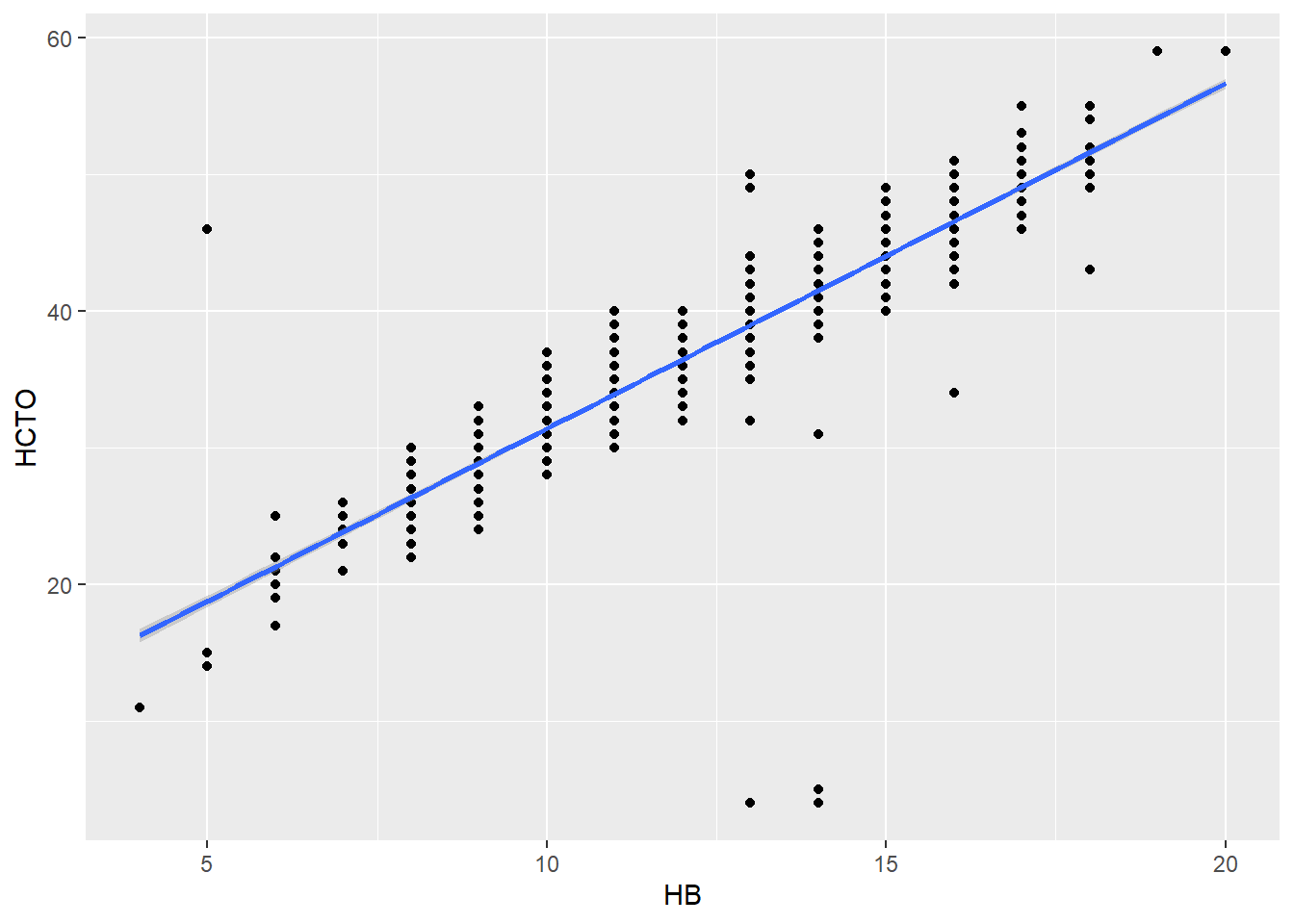The R-squared coefficient returned by lm function (0.8326), tells us that the model is well fitted and we can explain an 83% of the variability with this model.

We can try to analyze better our model to check if it changes depending on whether the patient suffer from malnutrition or no.

First, we split the dataset (patients with and without malnutrition)

data_des=data[data$DESNUTR=="si",] data_no_des=data[data$DESNUTR=="no",]

Fitting the model with patients that are suffering malnutrition.

regrlineal =lm(formula=HCTO ~ HB, data=data_des)
summary(regrlineal)
##
## Call:
## lm(formula = HCTO ~ HB, data = data_des)
##
## Residuals:
##     Min      1Q  Median      3Q     Max
## -3.2880 -1.1145 -0.1340  0.7651  5.1739
##
## Coefficients:
##             Estimate Std. Error t value Pr(>|t|)
## (Intercept)  3.73044    0.70881   5.263 1.11e-06 ***
## HB           2.71151    0.06087  44.543  < 2e-16 ***
## ---
## Signif. codes:  0 '***' 0.001 '**' 0.01 '*' 0.05 '.' 0.1 ' ' 1
##
## Residual standard error: 1.519 on 82 degrees of freedom
## Multiple R-squared:  0.9603, Adjusted R-squared:  0.9598
## F-statistic:  1984 on 1 and 82 DF,  p-value: < 2.2e-16

In this case the resultant equation is:

$HCTO= 3.73044 + 2.71151 * HB$

As we can see this model fits better than the previous one with a very high R-squared coefficient (0.9603).

Let’s check it visually:

ggplot(data_des,aes(x=HB,y=HCTO))+geom_point()+ geom_smooth(method="lm")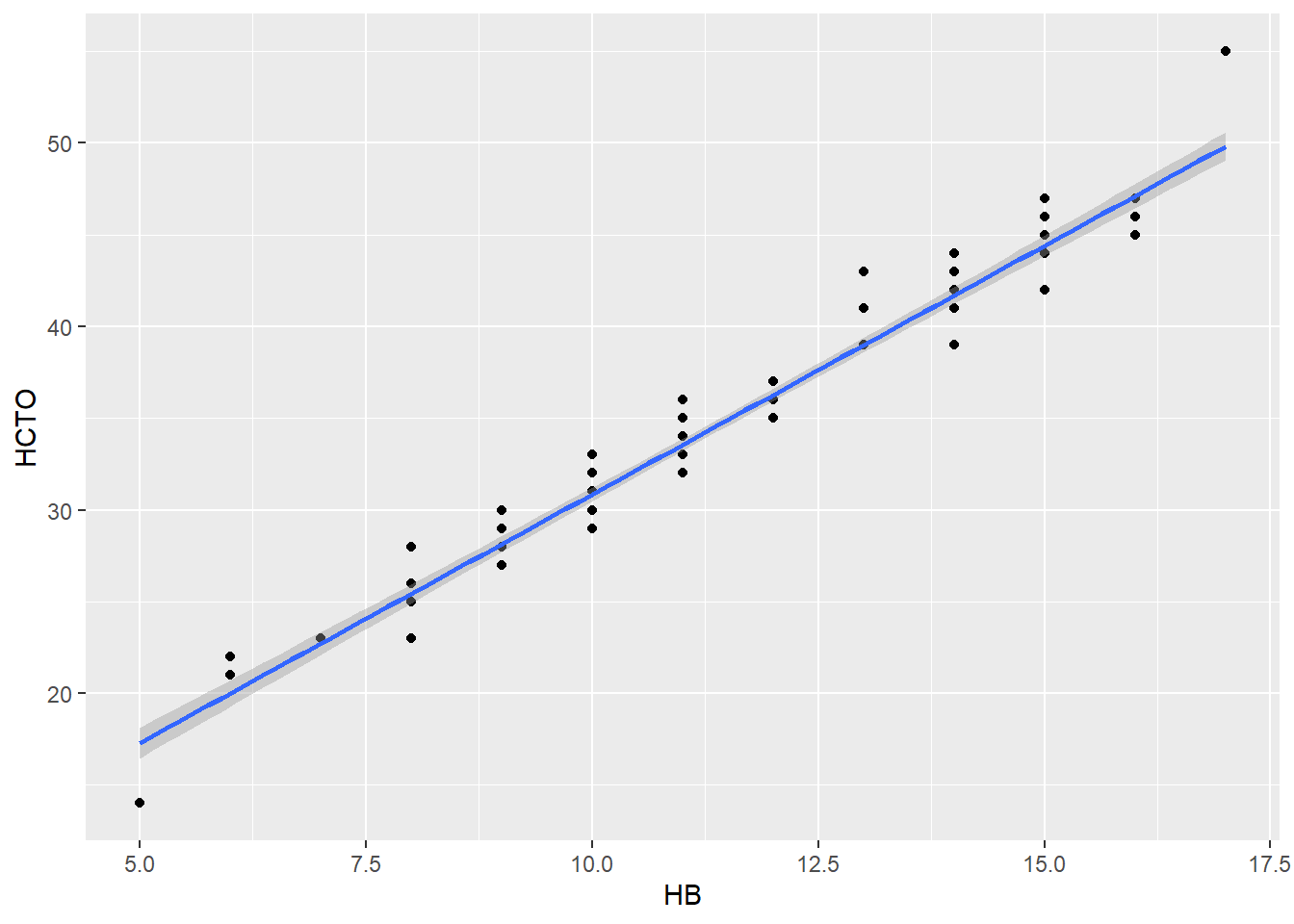Let’s repeat the model with the patients without malnutrition.

regrlineal =lm(formula=HCTO ~ HB, data=data_no_des)
summary(regrlineal)
##
## Call:
## lm(formula = HCTO ~ HB, data = data_no_des)
##
## Residuals:
##     Min      1Q  Median      3Q     Max
## -37.508  -1.011  -0.005   1.001  27.018
##
## Coefficients:
##             Estimate Std. Error t value Pr(>|t|)
## (Intercept)  6.46833    0.36945   17.51   <2e-16 ***
## HB           2.50282    0.02756   90.83   <2e-16 ***
## ---
## Signif. codes:  0 '***' 0.001 '**' 0.01 '*' 0.05 '.' 0.1 ' ' 1
##
## Residual standard error: 2.428 on 1850 degrees of freedom
## Multiple R-squared:  0.8168, Adjusted R-squared:  0.8167
## F-statistic:  8249 on 1 and 1850 DF,  p-value: < 2.2e-16

The resultant equation is:

$HCTO= 6.46833 + 2.50282 * HB$

For this type of patients the model does not fit so well, we can explain only 81% of the variability.

ggplot(data_no_des,aes(x=HB,y=HCTO))+geom_point()+ geom_smooth(method="lm")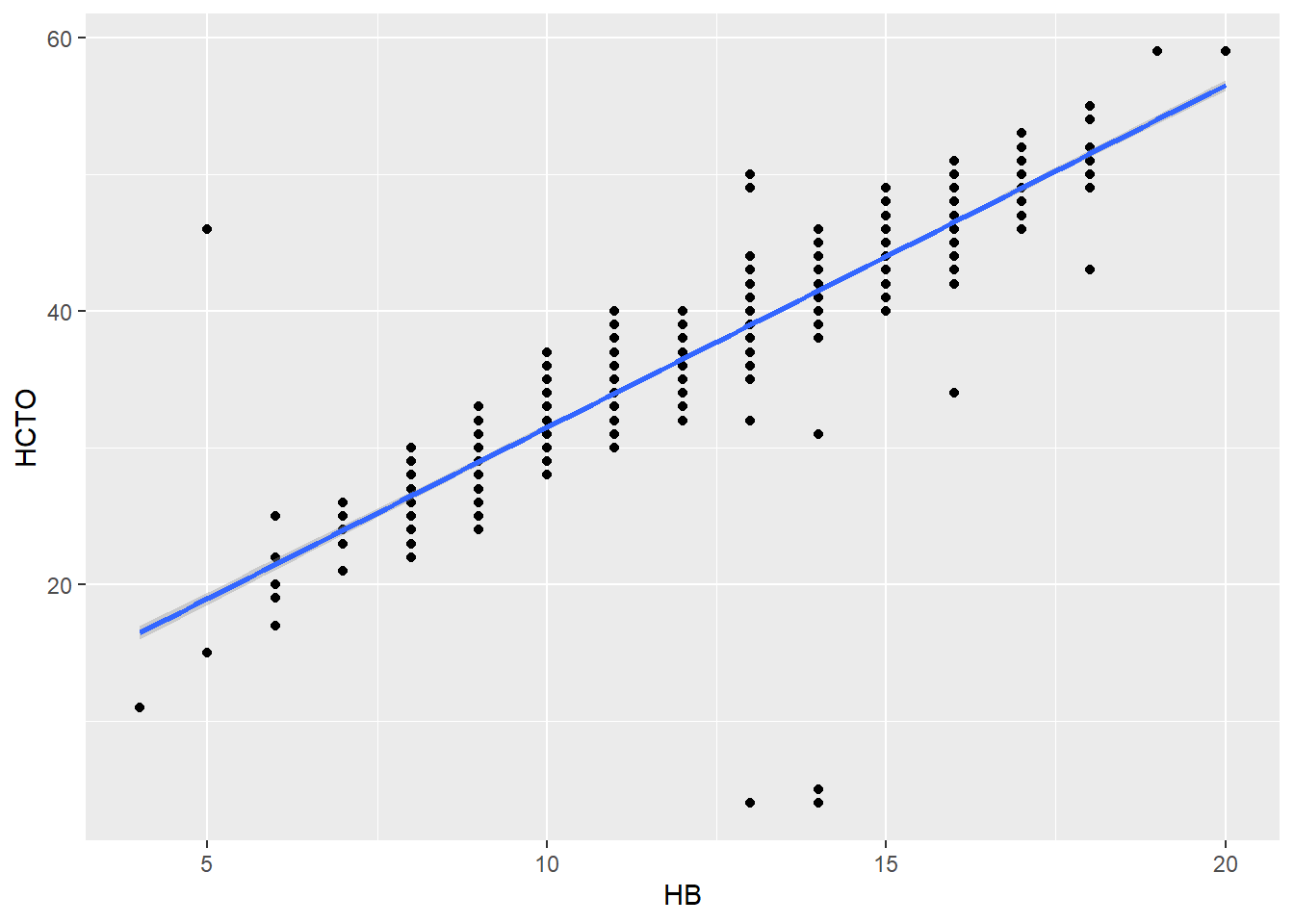We can conclude that there is not a big difference between patients with or without malnutrition, the model fits better in patients with malnutrition but according to the graphics, the linear relationship is similar in both types of patients.

## Multiple linear regression. Quantitative variables

Let’s fit a model with two independent variables, hematocrit and age (HTCO and EDAD)

regrlineal =lm(formula=HCTO ~ HB+EDAD, data=data)
summary(regrlineal)
##
## Call:
## lm(formula = HCTO ~ HB + EDAD, data = data)
##
## Residuals:
##     Min      1Q  Median      3Q     Max
## -37.739  -1.057   0.010   1.172  27.467
##
## Coefficients:
##             Estimate Std. Error t value Pr(>|t|)
## (Intercept) 5.127968   0.409978  12.508  < 2e-16 ***
## HB          2.551765   0.026293  97.049  < 2e-16 ***
## EDAD        0.012667   0.002765   4.581 4.93e-06 ***
## ---
## Signif. codes:  0 '***' 0.001 '**' 0.01 '*' 0.05 '.' 0.1 ' ' 1
##
## Residual standard error: 2.387 on 1933 degrees of freedom
## Multiple R-squared:  0.8344, Adjusted R-squared:  0.8342
## F-statistic:  4869 on 2 and 1933 DF,  p-value: < 2.2e-16

The resultant equation is:

$HCTO= 5.127968 + 2.551765 * HB + 0.012667* EDAD$

We see that the coefficient of determination R2 (adjusted) in this case is 0.8342, which is quite similar to the adjusted R2 value of section 1.1 a), this means that this model explains somewhat more of the variation in the data than the previous one, but it is not a substantial improvement.

As for the coefficients obtained, we can say that the first coefficient (5.127968) represents the Y estimate when the other variables are zero. In this case it does not make much sense since age does not take the value zero, nor does hemoglobin, making this parameter difficult to interpret.

The second parameter (2.551765) represents the increase of the variable HCTO when hemoglobin HB increases 1 unit, keeping age constant, in the same way, the third parameter (0.012667) represents the increase of the variable HCTO when AGE increases 1 unit keeping HB constant. As we can see the variation of Y with respect to the variation of age is very small, much smaller than when hemoglobin (HB) increases.

Finally, let us look at the graph.

ggplot(data,aes(y=HCTO ,x=HB ,color=EDAD))+geom_point()+stat_smooth(method="lm",se=FALSE)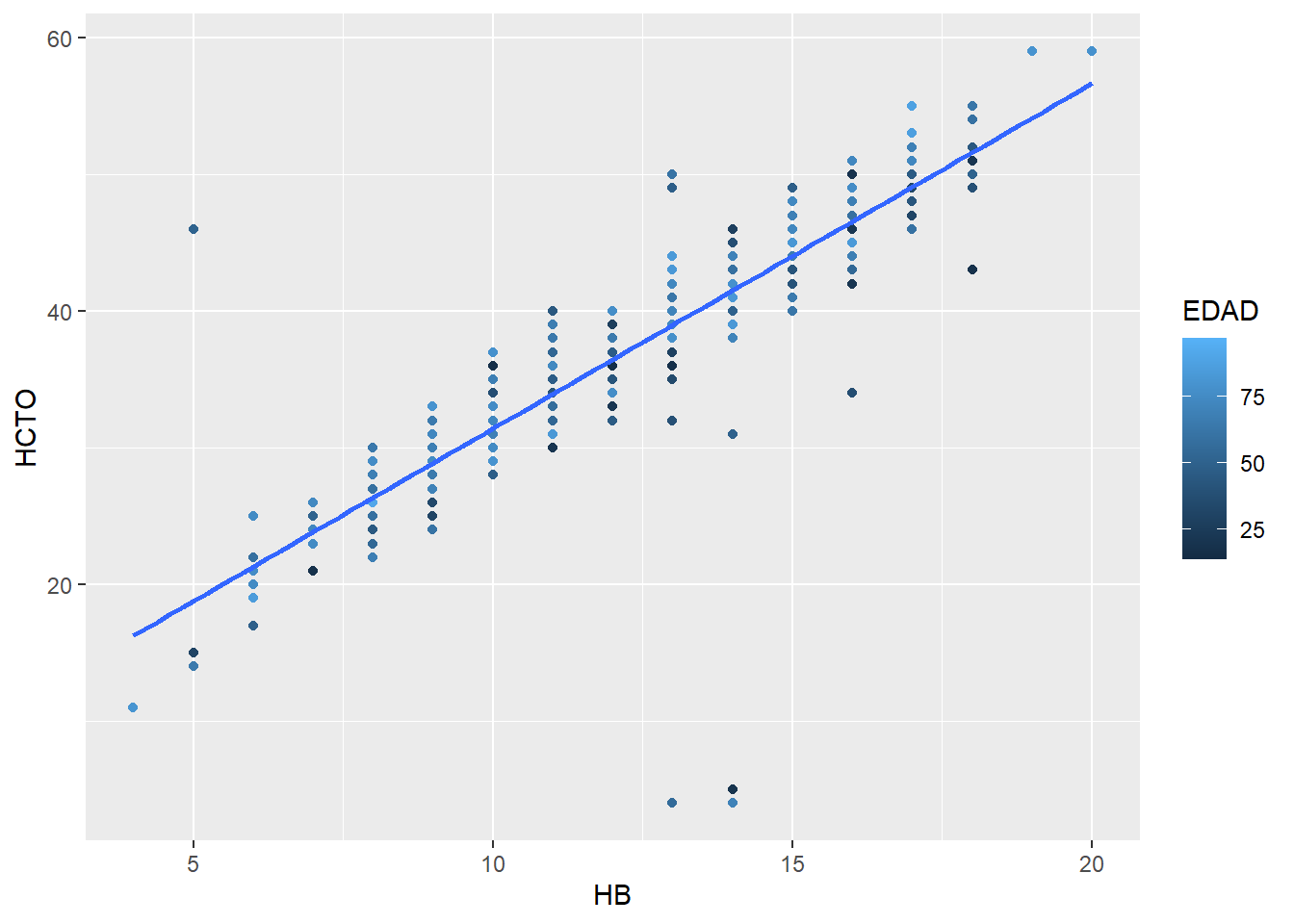## Multiple linear regression model.Quantitative and Qualitative Variables.

We want to know to what extent hematocrit is related to hemoglobin and age, depending on whether or not patients have post-surgical infection. Apply a multiple linear regression model and explain the result.

regrlineal1 =lm(formula=HCTO ~ HB+EDAD+INFEC, data=data)
summary(regrlineal1)
##
## Call:
## lm(formula = HCTO ~ HB + EDAD + INFEC, data = data)
##
## Residuals:
##     Min      1Q  Median      3Q     Max
## -37.614  -1.057   0.012   1.180  27.407
##
## Coefficients:
##              Estimate Std. Error t value Pr(>|t|)
## (Intercept)  5.184732   0.412829  12.559  < 2e-16 ***
## HB           2.548186   0.026470  96.266  < 2e-16 ***
## EDAD         0.013085   0.002788   4.693 2.88e-06 ***
## INFECsi     -0.161460   0.138660  -1.164    0.244
## ---
## Signif. codes:  0 '***' 0.001 '**' 0.01 '*' 0.05 '.' 0.1 ' ' 1
##
## Residual standard error: 2.386 on 1932 degrees of freedom
## Multiple R-squared:  0.8345, Adjusted R-squared:  0.8342
## F-statistic:  3247 on 3 and 1932 DF,  p-value: < 2.2e-16

In this case the variable INFEC is divided into 2 dummy variables according to the possible values of the variable. The formulas we would have are the following:

If the patient was not infected:

$HCTO= 5.184732 + 2.548186 * HB + 0.013085 * EDAD$

If the patient was infected: $HCTO= 3.022740 - 0.161460 + 2.548186 * HB + 0.013085 * EDAD$

As we have 2 dummy variables, we have another new intersection value if the value of INFEC is yes, which is added to the original intersection value (this absorbs the cases where INFEC is no).

In this case we obtain a fairly good goodness of fit since the coefficient of determination R2 is (0.8345), so the model has a goodness of fit similar to that obtained in the first section.

We see the scatter plot. In this case, as there is such a short distance between both lines (0.16), they appear almost overlapped in the plot.

eq1=function(x){coef(regrlineal1)+coef(regrlineal1)*x}
eq2=function(x){coef(regrlineal1)+coef(regrlineal1)+coef(regrlineal1)*x}
stat_function(fun=eq2,geom="line",color=scales::hue_pal()(2))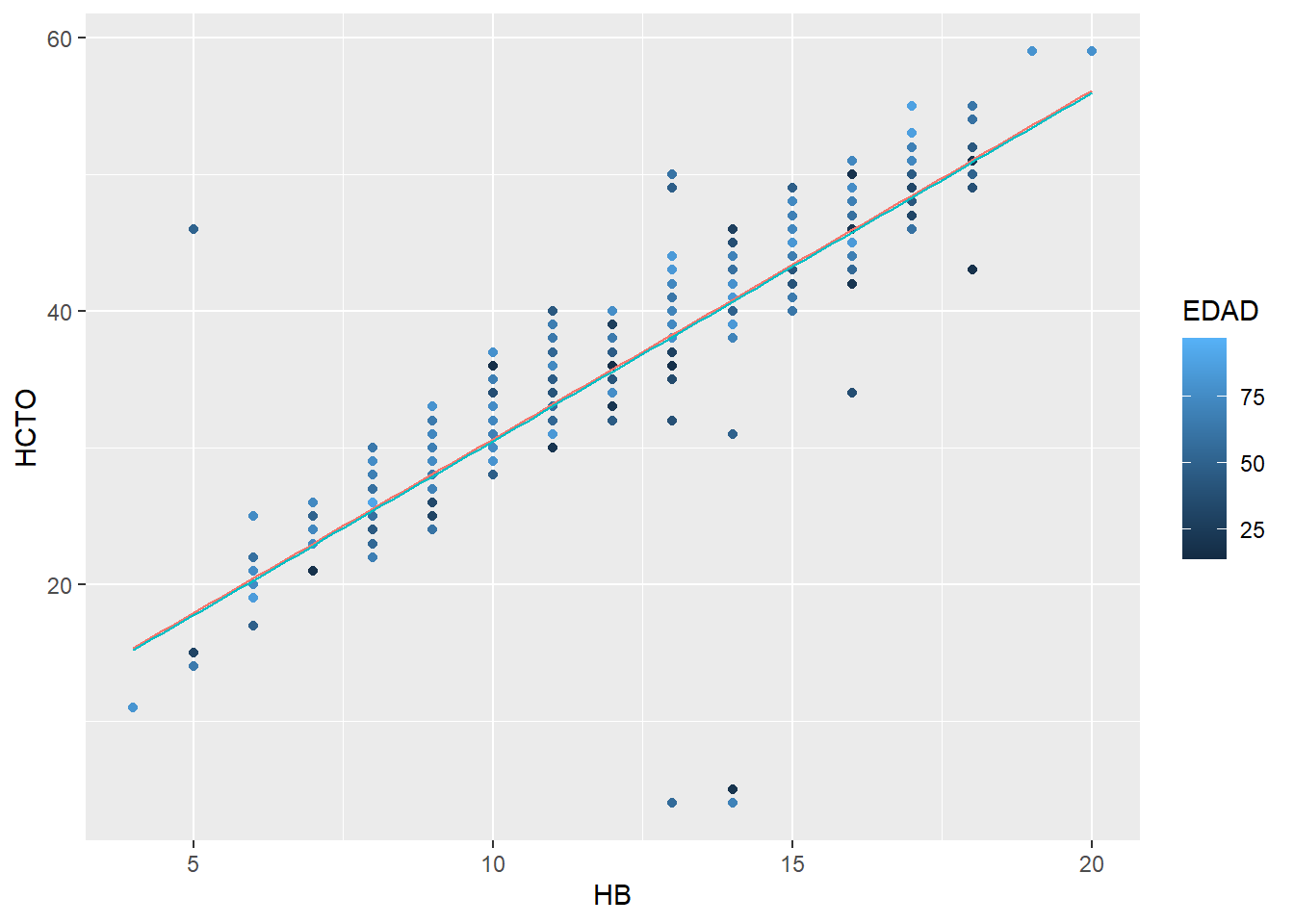Let’s repeat the study,taking only those patients whose hematocrit is < 37. Compare with the previous model and draw conclusions.

First, we get the rows in the dataset with HCTO < 37

data_htco=data[data$HCTO<37,] Let’s calculate the linear regression: regrlineal2 =lm(formula=HCTO ~ HB+EDAD+INFEC, data=data_htco) summary(regrlineal2) ## ## Call: ## lm(formula = HCTO ~ HB + EDAD + INFEC, data = data_htco) ## ## Residuals: ## Min 1Q Median 3Q Max ## -34.704 -0.845 0.303 1.393 6.236 ## ## Coefficients: ## Estimate Std. Error t value Pr(>|t|) ## (Intercept) 9.723567 1.127075 8.627 <2e-16 *** ## HB 2.019812 0.087761 23.015 <2e-16 *** ## EDAD 0.016232 0.007592 2.138 0.033 * ## INFECsi -0.433599 0.314835 -1.377 0.169 ## --- ## Signif. codes: 0 '***' 0.001 '**' 0.01 '*' 0.05 '.' 0.1 ' ' 1 ## ## Residual standard error: 3.216 on 496 degrees of freedom ## Multiple R-squared: 0.5292, Adjusted R-squared: 0.5264 ## F-statistic: 185.8 on 3 and 496 DF, p-value: < 2.2e-16 The resultant equations are: If the patient was not infected: $HCTO= 9.723567 + 2.019812 * HB + 0.016232 * EDAD$ If the patient was infeted. $HCTO= 9.723567 - 0.433599 + 2.019812 * HB + 0.016232 * EDAD$ We see a much lower goodness of fit (R2=0.5292), in this case the model only explains approximately half of the variability of the data. This means that for patients with hematocrit less than 37 there is not such a clear linear relationship between the variables studied. eq1=function(x){coef(regrlineal2)+coef(regrlineal2)*x} eq2=function(x){coef(regrlineal2)+coef(regrlineal2)+coef(regrlineal2)*x} ggplot(data_htco,aes(y=HCTO ,x=HB ,color=EDAD))+geom_point()+stat_function(fun=eq1,geom="line",color=scales::hue_pal()(2))+ stat_function(fun=eq2,geom="line",color=scales::hue_pal()(2))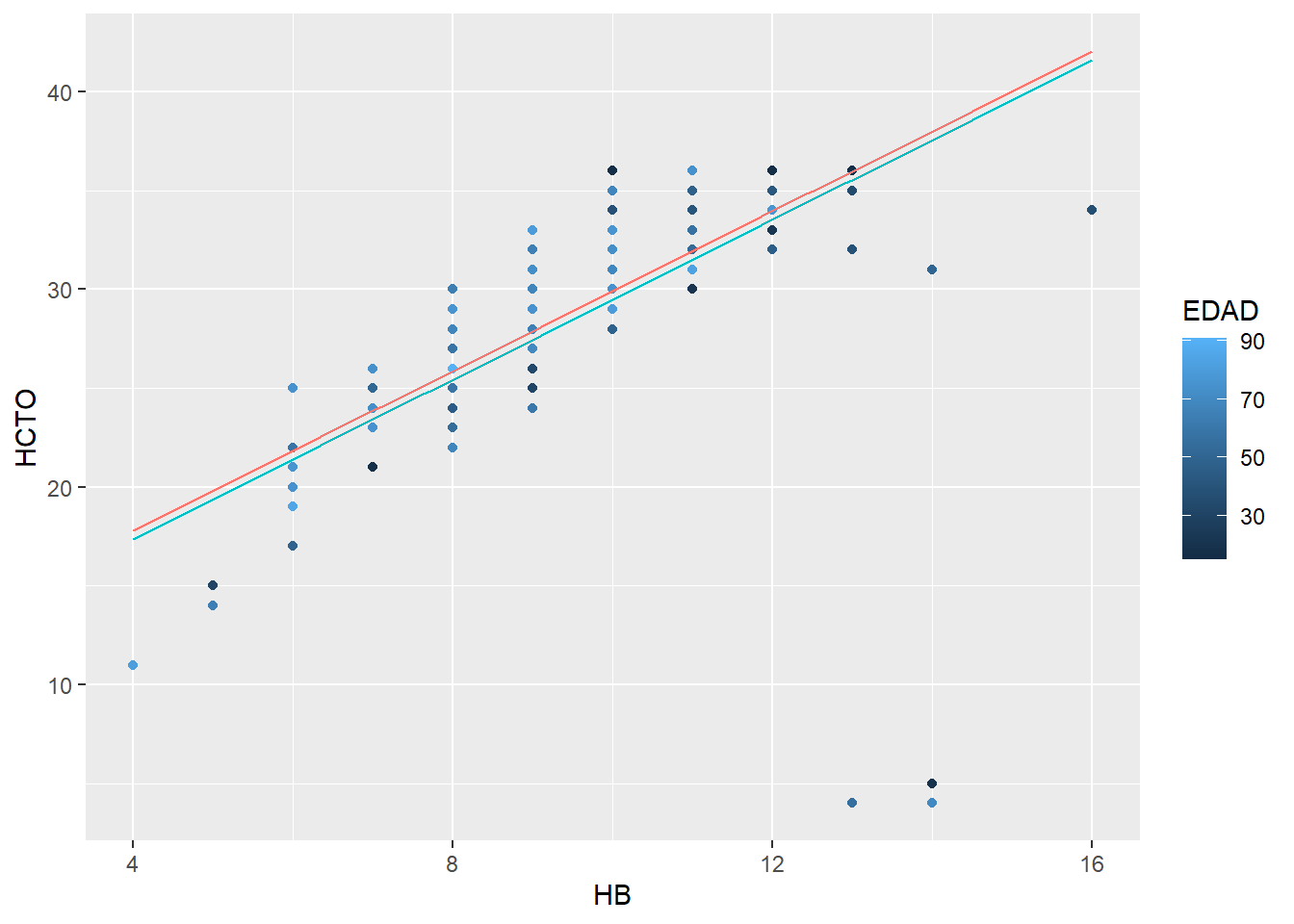## Predictive model Let’s fit a predictive model with the previous linear models We are going to make a prediction for a 60-year-old patient, with post-surgical infection and a hemoglobin value of 10. Let’s create a dataset with the new patient data. new.data<-data.frame("HB"=c(10),"EDAD"=c(60),"INFEC"=c("si")) new.data ## HB EDAD INFEC ## 1 10 60 si #Realizamos la predicción predict(regrlineal1,newdata=new.data,interval="confidence") ## fit lwr upr ## 1 31.29021 31.01741 31.563 In this case we have a prediction of 31.29021, with a confidence interval [31.01741,31.563] of 95%. Let’s make a prediction with the other model. predict(regrlineal2,newdata=new.data,interval="confidence") ## fit lwr upr ## 1 30.46201 29.95307 30.97095 The prediction obtained in this case is 30.46201. The confidence interval is [29.95307,30.97095], in this case we observe how for this second model, where the goodness of fit was lower, the confidence interval for the prediction is doubled with respect to the previous model. # Logistic regression. ## OR (Odds Ratio) estimation Let’s identify the risk factors for post-surgical infection. Therefore, the probability that a patient may or may not have an infection will be evaluated, depending on whether or not he/she presents certain characteristics. First we perform a univariate analysis of possible risk factors associated with post-surgical infection. We start performing a chi-squared test to check if the variables are independent. Let’s start with the relationship between post-surgical infection and diabetes. DIABETES To perform the chi-square test we create the contingency table. tbl=table(data$INFEC,data$DIABETES) tbl ## ## no si ## no 1475 77 ## si 350 34 We run the chi-square test. We see that the default formula applies the Yates correction. chisq.test(tbl) ## ## Pearson's Chi-squared test with Yates' continuity correction ## ## data: tbl ## X-squared = 7.926, df = 1, p-value = 0.004873 The p-value is well below 0.05, so we must reject the null hypothesis, in this case the variables are not independent. We calculate the odds ratio, by hand and then with the help of the questionr library. # odds = (N00/N01)*(N11/N10) odds=(1475/77)*(34/350) odds ##  1.860853 library(questionr) odds.ratio(tbl) ## OR 2.5 % 97.5 % p ## Fisher's test 1.8601 1.1839 2.8724 0.00472 ** ## --- ## Signif. codes: 0 '***' 0.001 '**' 0.01 '*' 0.05 '.' 0.1 ' ' 1 In this case we have an odds ratio of 1.8608, which means that post-surgical infection and diabetes are related, that is, the presence of one favors the presence of the other. By obtaining a P-Value < 0.05 we can determine that this odds value is significant. MALNUTRITION tbl=table(data$INFEC,data$DESNUTR) tbl ## ## no si ## no 1506 46 ## si 346 38 Ejecutamos el test chi-cuadrado. chisq.test(tbl) ## ## Pearson's Chi-squared test with Yates' continuity correction ## ## data: tbl ## X-squared = 33.988, df = 1, p-value = 5.547e-09 In this case the p-value is quite small which means that we must reject the null hypothesis, again we determine that the variables are not independent. Let’s calculate the odds ratio. # odds = (N00/N01)*(N11/N10) odds=(1506/46)*(38/346) odds ##  3.595627 library(questionr) odds.ratio(tbl) ## OR 2.5 % 97.5 % p ## Fisher's test 3.5925 2.2370 5.7412 6.296e-08 *** ## --- ## Signif. codes: 0 '***' 0.001 '**' 0.01 '*' 0.05 '.' 0.1 ' ' 1 In this case we have an odds ratio of 3.5956, higher than the previous one, which means that the presence of malnutrition is more related to post-surgical infection than diabetes, this makes sense given the small p-value obtained in the chi-square test that indicated a strong relationship between these variables. OBESITY Let’s perform the calculation with obesity. tbl=table(data$INFEC,data$OBES) tbl ## ## no si ## no 1314 238 ## si 290 94 Now, the chi-square test chisq.test(tbl) ## ## Pearson's Chi-squared test with Yates' continuity correction ## ## data: tbl ## X-squared = 17.478, df = 1, p-value = 2.906e-05 Again, we obtain a rather small p-value, which indicates that there is also some relationship between these variables. We calculate the odds ratio in both ways, first manually and then with the odds.ratio function. # odds = (N00/N01)*(N11/N10) odds=(1314/238)*(94/290) odds ##  1.789568 library(questionr) odds.ratio(tbl) ## OR 2.5 % 97.5 % p ## Fisher's test 1.7891 1.3489 2.3612 4.1e-05 *** ## --- ## Signif. codes: 0 '***' 0.001 '**' 0.01 '*' 0.05 '.' 0.1 ' ' 1 In this case we have an odds ratio of 1.77891, again we see how the p-value indicates significance in the relationship between the variables. AGE In this case we cannot run the chi-square test since it is for categorical variables. To calculate the odds ratio of age we cannot do it by the contingency table since it is a continuous variable, we will use a logistic regression model. (logit) logit<-glm(INFEC ~ EDAD, data=data, family="binomial") summary(logit) ## ## Call: ## glm(formula = INFEC ~ EDAD, family = "binomial", data = data) ## ## Deviance Residuals: ## Min 1Q Median 3Q Max ## -0.9565 -0.7284 -0.6041 -0.4646 2.1702 ## ## Coefficients: ## Estimate Std. Error z value Pr(>|z|) ## (Intercept) -2.568056 0.188421 -13.629 < 2e-16 *** ## EDAD 0.020858 0.003061 6.813 9.53e-12 *** ## --- ## Signif. codes: 0 '***' 0.001 '**' 0.01 '*' 0.05 '.' 0.1 ' ' 1 ## ## (Dispersion parameter for binomial family taken to be 1) ## ## Null deviance: 1928.7 on 1935 degrees of freedom ## Residual deviance: 1879.0 on 1934 degrees of freedom ## AIC: 1883 ## ## Number of Fisher Scoring iterations: 4 In this case the coefficients are the logarithm of the odds ratio, so we can obtain the odds ratio with the following instruction. exp(coefficients(logit)) ## (Intercept) EDAD ## 0.07668446 1.02107746 This means that an increase of one year in age increases the probability of post-surgical infection by 2%. HEMATOCRIT logit<-glm(INFEC ~ HCTO, data=data, family="binomial") summary(logit) ## ## Call: ## glm(formula = INFEC ~ HCTO, family = "binomial", data = data) ## ## Deviance Residuals: ## Min 1Q Median 3Q Max ## -1.4247 -0.6792 -0.6136 -0.5390 2.2838 ## ## Coefficients: ## Estimate Std. Error z value Pr(>|z|) ## (Intercept) 0.790069 0.363718 2.172 0.0298 * ## HCTO -0.056296 0.009371 -6.008 1.88e-09 *** ## --- ## Signif. codes: 0 '***' 0.001 '**' 0.01 '*' 0.05 '.' 0.1 ' ' 1 ## ## (Dispersion parameter for binomial family taken to be 1) ## ## Null deviance: 1928.7 on 1935 degrees of freedom ## Residual deviance: 1892.6 on 1934 degrees of freedom ## AIC: 1896.6 ## ## Number of Fisher Scoring iterations: 4 We calculate the odds as in the previous case exp(coefficients(logit)) ## (Intercept) HCTO ## 2.2035493 0.9452588 These odds indicate that a 1 point increase in hematocrit decreases the chances of surgical infection by 6%. Let’s check the relationship between the variables infection and operation type. We can apply the logit model on the categories, in this case we obtain the probabilities for each TIP_OPER category separately. logit<-glm(INFEC ~ TIP_OPER, data=data, family="binomial") summary(logit) ## ## Call: ## glm(formula = INFEC ~ TIP_OPER, family = "binomial", data = data) ## ## Deviance Residuals: ## Min 1Q Median 3Q Max ## -1.0733 -0.6556 -0.3593 -0.3593 2.3548 ## ## Coefficients: ## Estimate Std. Error z value Pr(>|z|) ## (Intercept) -0.9951 0.1498 -6.641 3.12e-11 *** ## TIP_OPERlimpia -1.7130 0.2148 -7.973 1.55e-15 *** ## TIP_OPERpot_cont -0.4330 0.1804 -2.401 0.0164 * ## TIP_OPERsucia 0.7452 0.1842 4.045 5.22e-05 *** ## --- ## Signif. codes: 0 '***' 0.001 '**' 0.01 '*' 0.05 '.' 0.1 ' ' 1 ## ## (Dispersion parameter for binomial family taken to be 1) ## ## Null deviance: 1928.7 on 1935 degrees of freedom ## Residual deviance: 1710.2 on 1932 degrees of freedom ## AIC: 1718.2 ## ## Number of Fisher Scoring iterations: 5 levels(data$TIP_OPER)
##  "contam"   "limpia"   "pot_cont" "sucia"

Let’s find the odds ratio:

exp(coefficients(logit))
##      (Intercept)   TIP_OPERlimpia TIP_OPERpot_cont    TIP_OPERsucia
##        0.3696970        0.1803279        0.6485476        2.1068457

We have the odds of suffering a post-surgical infection according to the type of operation. The contaminated type is absorbed within “(Intercept)”, we see how there is a much higher chance of infection with a “dirty” type of operation than with a “clean” type of operation.

## Logistic regression model

Let’s check if having diabetes is a risk factor for infection in the operation.

Let’s fit the model

logit<-glm(INFEC ~ DIABETES, data=data, family="binomial")
summary(logit)
##
## Call:
## glm(formula = INFEC ~ DIABETES, family = "binomial", data = data)
##
## Deviance Residuals:
##     Min       1Q   Median       3Q      Max
## -0.8552  -0.6526  -0.6526  -0.6526   1.8174
##
## Coefficients:
##             Estimate Std. Error z value Pr(>|z|)
## (Intercept) -1.43848    0.05946 -24.194  < 2e-16 ***
## DIABETESsi   0.62104    0.21432   2.898  0.00376 **
## ---
## Signif. codes:  0 '***' 0.001 '**' 0.01 '*' 0.05 '.' 0.1 ' ' 1
##
## (Dispersion parameter for binomial family taken to be 1)
##
##     Null deviance: 1928.7  on 1935  degrees of freedom
## Residual deviance: 1920.9  on 1934  degrees of freedom
## AIC: 1924.9
##
## Number of Fisher Scoring iterations: 4

Let’s calculate the probabilities.

exp(coefficients(logit))
## (Intercept)  DIABETESsi
##   0.2372881   1.8608534

In this case we see that both p-values are less than 0.05 so diabetes does seem to be a risk factor to take into account. In this case we see that the presence of diabetes makes the presence of a post-surgical infection more probable.

In relation to the previous section, we see that the odds obtained with this model are very similar to the odds obtained with the contingency table obtained previously.

We add the explanatory variables age and hematocrit to the previous model. Evaluate whether any of the regressors has a significant influence (p-value of the individual contrast lower than 5%).

logit<-glm(INFEC ~ DIABETES+EDAD+HCTO, data=data, family="binomial")
summary(logit)
##
## Call:
## glm(formula = INFEC ~ DIABETES + EDAD + HCTO, family = "binomial",
##     data = data)
##
## Deviance Residuals:
##     Min       1Q   Median       3Q      Max
## -1.2563  -0.6970  -0.5836  -0.4508   2.3125
##
## Coefficients:
##              Estimate Std. Error z value Pr(>|z|)
## (Intercept) -0.631955   0.433490  -1.458    0.145
## DIABETESsi   0.291478   0.220867   1.320    0.187
## EDAD         0.017845   0.003142   5.679 1.35e-08 ***
## HCTO        -0.045983   0.009490  -4.845 1.26e-06 ***
## ---
## Signif. codes:  0 '***' 0.001 '**' 0.01 '*' 0.05 '.' 0.1 ' ' 1
##
## (Dispersion parameter for binomial family taken to be 1)
##
##     Null deviance: 1928.7  on 1935  degrees of freedom
## Residual deviance: 1853.4  on 1932  degrees of freedom
## AIC: 1861.4
##
## Number of Fisher Scoring iterations: 4

In this case we see that the variation of age and hematocrit are more significant than diabetes, since the p-value for diabetes is > 0.05 while for the other 2 is lower. Therefore, the influential regressors in this case are age and hematocrit.

## Model improvement.

Let’s repeat the model but categorizing

Let’s categorize the continuous variables, we create two new categorized variables.

Age and HTCO

data$EDADCAT<-cut(data$EDAD, breaks=c(0,65,max(data$EDAD)),labels=c("<65",">=65"),right=FALSE) data$HCTOCAT<-cut(data$HCTO, breaks=c(0,37,max(data$HCTO)),labels=c("<37",">=37"),right=FALSE)
str(data)
## 'data.frame':    1936 obs. of  18 variables:
##  $EDAD : int 59 65 70 55 56 65 70 80 53 77 ... ##$ SEXO    : Factor w/ 2 levels "mujer","varón": 1 1 1 1 1 1 1 1 1 1 ...
##  $PATOL : Factor w/ 4 levels "inflam","neo",..: 1 3 2 3 4 3 2 3 2 2 ... ##$ TIP_OPER: Factor w/ 4 levels "contam","limpia",..: 4 3 1 3 1 3 3 3 3 3 ...
##  $ALB : Factor w/ 8 levels "1","2","3","3.9",..: 5 5 NA 5 NA 3 5 3 5 3 ... ##$ HB      : int  13 12 14 12 13 11 8 12 10 12 ...
##  $HCTO : int 38 36 39 38 41 35 28 36 29 37 ... ##$ LEUCOS  : int  19090 6190 8200 4800 5550 5840 7400 4130 4810 9590 ...
##  $LINFOPCT: int 12 33 23 51 40 48 20 17 20 13 ... ##$ HEMAT   : int  4 4 5 4 5 4 4 4 3 4 ...
##  $GLUC : int 68 79 87 92 92 93 96 97 99 99 ... ##$ OBES    : Factor w/ 2 levels "no","si": 2 2 1 2 1 2 1 1 1 1 ...
##  $DESNUTR : Factor w/ 2 levels "no","si": 1 1 2 1 1 1 1 1 2 1 ... ##$ DIABETES: Factor w/ 2 levels "no","si": 2 2 2 2 2 2 2 2 2 2 ...
##  $INFEC : Factor w/ 2 levels "no","si": 2 1 1 2 2 1 1 1 1 2 ... ##$ GLUC_4  : Factor w/ 4 levels "(115-138]","<70",..: 2 4 4 4 4 4 4 4 4 4 ...
##  $EDADCAT : Factor w/ 2 levels "<65",">=65": 1 2 2 1 1 2 2 2 1 2 ... ##$ HCTOCAT : Factor w/ 2 levels "<37",">=37": 2 1 2 2 2 1 1 1 1 2 ...

Fitting the model.

logit<-glm(INFEC ~ DIABETES+EDADCAT+HCTOCAT, data=data, family="binomial")
summary(logit)
##
## Call:
## glm(formula = INFEC ~ DIABETES + EDADCAT + HCTOCAT, family = "binomial",
##     data = data)
##
## Deviance Residuals:
##     Min       1Q   Median       3Q      Max
## -1.0930  -0.6829  -0.5307  -0.5307   2.0149
##
## Coefficients:
##             Estimate Std. Error z value Pr(>|z|)
## (Intercept)  -1.1206     0.1187  -9.442  < 2e-16 ***
## DIABETESsi    0.3666     0.2210   1.659   0.0972 .
## EDADCAT>=65   0.5522     0.1196   4.618 3.88e-06 ***
## HCTOCAT>=37  -0.7685     0.1232  -6.240 4.38e-10 ***
## ---
## Signif. codes:  0 '***' 0.001 '**' 0.01 '*' 0.05 '.' 0.1 ' ' 1
##
## (Dispersion parameter for binomial family taken to be 1)
##
##     Null deviance: 1924.1  on 1931  degrees of freedom
## Residual deviance: 1846.5  on 1928  degrees of freedom
##   (4 observations deleted due to missingness)
## AIC: 1854.5
##
## Number of Fisher Scoring iterations: 4
exp(coefficients(logit))
## (Intercept)  DIABETESsi EDADCAT>=65 HCTOCAT>=37
##   0.3260772   1.4428201   1.7369922   0.4637203

In this case we observe that the variables age and hematocrit continue to be significant, given their p-value, the difference in this case is that their influence on the dependent variable post-surgical infection is much greater than in the previous section. We observe that for an age >=65 the probabilities of suffering infection increase, in the case of hematocrit, being above 37 decreases the probabilities of suffering post-surgical infection.

Let’s add the malnutrition variable to the model.

logit<-glm(INFEC ~ DIABETES+EDADCAT+HCTOCAT+DESNUTR, data=data, family="binomial")
summary(logit)
##
## Call:
## glm(formula = INFEC ~ DIABETES + EDADCAT + HCTOCAT + DESNUTR,
##     family = "binomial", data = data)
##
## Deviance Residuals:
##     Min       1Q   Median       3Q      Max
## -1.3975  -0.6696  -0.5287  -0.5287   2.0183
##
## Coefficients:
##             Estimate Std. Error z value Pr(>|z|)
## (Intercept)  -1.2057     0.1223  -9.861  < 2e-16 ***
## DIABETESsi    0.3418     0.2217   1.542  0.12311
## EDADCAT>=65   0.5158     0.1206   4.276 1.90e-05 ***
## HCTOCAT>=37  -0.6913     0.1259  -5.489 4.04e-08 ***
## DESNUTRsi     0.8520     0.2367   3.599  0.00032 ***
## ---
## Signif. codes:  0 '***' 0.001 '**' 0.01 '*' 0.05 '.' 0.1 ' ' 1
##
## (Dispersion parameter for binomial family taken to be 1)
##
##     Null deviance: 1924.1  on 1931  degrees of freedom
## Residual deviance: 1834.1  on 1927  degrees of freedom
##   (4 observations deleted due to missingness)
## AIC: 1844.1
##
## Number of Fisher Scoring iterations: 4

In this case the model does improve, since we have a smaller residual deviation, and the AIC indicator is also smaller when the malnutrition variable is added.

## Prediction

According to the model in the previous section, let’s check the probability of post-surgical infection in a 50-year-old patient with diabetes, hematocrit concentration of 34, and no malnutrition?

new.data<-data.frame("DIABETES"=c("si"),"EDADCAT"=c("<65"),"HCTOCAT"=c("<37"),"DESNUTR"=c("no"))
new.data
##   DIABETES EDADCAT HCTOCAT DESNUTR
## 1       si     <65     <37      no
#Realizamos la predicción
predict(logit,newdata=new.data,type="response")
##         1
## 0.2965271

In this case, with the data entered in the model, the probability is 29.65%.

Discussion

According to the results obtained, we can see that when studied separately, all the variables have an influence on the probability of suffering a post-surgical infection (diabetes, malnutrition, obesity, age and hematocrit); however, when we study the combined influence of all the variables, we see that diabetes is no longer a significant factor.

From the separate study of all the variables, we can conclude that malnutrition and the type of operation are the variables that have the greatest influence on the risk of suffering post-surgical infection, since the odds are very high and the probabilities vary considerably in the case of malnutrition or a dirty operation.

Finally, we have seen how age and hematocrit influence the risk of infection, treated as continuous variables we see how the risk of infection gradually increases in relation to an increase in age or decrease in hematocrit, this is much clearer when we categorize these variables and carry out the study by ranges.